# java方法

``````public static void main(String[] args) {
//请计算10和20的和
int a = 10;
int b = 20;
int c = a + b;
System.out.println(a + "+" + b + "=" + c);
//请计算666和888的和
int x = 666;
int y = 888;
int z = x + y;
System.out.println(x + "+" + y + "=" + z);
//请计算888和999的和
int m = 888;
int n = 999;
int e = m + n;
System.out.println(m + "+" + n + "=" + e);
}``````

``````public static void main(String[] args) {
//调用求和方法计算10和20的和
sumInt(10 , 20);
//调用求和方法计算666和888的和
sumInt(666 , 888);
//调用求和方法计算888和999的和
sumInt(888 , 999);
}
//专门负责求和的方法
public static void sumInt(int a , int b){
int c = a + b;
System.out.println(a + "+" + b + "=" + c);
}``````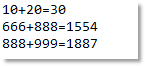7-1：求和结果

``````[修饰符列表] 返回值类型 方法名(形式参数列表){

}

public static void sumInt(int a , int b){
int c = a + b;
System.out.println(a + "+" + b + "=" + c);
}
public static是修饰符列表；
void是返回值类型；
sumInt是方法名；
(int a , int b)是形式参数列表，简称形参，每一个形参都是局部变量；

public static int sumInt(int a , int b){
int c = a + b;
return c;
}

• [修饰符列表]，此项是可选项，不是必须的，目前大家统一写成public static，后面的课程会详细讲解。

• 返回值类型，此项可以是java语言当中任何一种数据类型，包括基本数据类型，也包括所有的引用数据类型，当然，如果一个方法执行结束之后不准备返回任何数据，则返回值类型必须写void。返回值类型例如：byte,short,int,long,float,double,boolean,char,String,void等。

• 形式参数列表(int a, int b)，此项又被称为形参，其实每一个形参都是“局部变量”，形参的个数为0~N个，如果是多个参数，则采用半角“,”进行分隔，形参中起决定性作用的是参数的数据类型，参数名就是变量名，变量名是可以修改的，也就是说(int a , int b)也可以写成(int x , int y)

​​​​​​​

• 方法体，由一对儿大括号括起来，在形参的后面，这个大括号当中的是实现功能的核心代码，方法体由java语句构成，方法体当中的代码只能遵循自上而下的顺序依次逐行执行，不能跳行执行，核心代码在执行过程中如果需要外部提供数据，则通过形参进行获取。

​​​​​​​

``````public class MethodTest {
public static void main(String[] args) {
MethodTest.sumInt(100, 200);
MethodTest.sumDouble(1.0, 2.0);
}
public static void sumInt(int x , int y){
System.out.println(x + "+" + y + "=" + (x + y));
}
public static void sumDouble(double a , double b){
System.out.println(a + "+" + b + "=" + (a + b));
}
}``````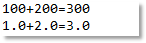7-2：方法如何调用

``````public class MethodTest03 {
public static void main(String[] args) {
sumInt(100, 200); //“类名.”可以省略
sumDouble(1.0, 2.0);//“类名.”可以省略
//doOther(); //编译报错
Other.doOther(); //“类名.”不能省略
}
public static void sumInt(int x , int y){
System.out.println(x + "+" + y + "=" + (x + y));
}
public static void sumDouble(double a , double b){
System.out.println(a + "+" + b + "=" + (a + b));
}
}``````
``````public class Other{
public static void doOther(){
System.out.println("Other doOther...");
}
}``````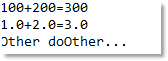7-3：“类名.”什么时候可以省略

java语言中方法的返回值类型可以是任何一种数据类型，包括基本数据类型，也包括引用数据类型，例如：byte,short,int,long,float,double,boolean,char,String,Student（自定义类）等。当然，如果这个方法在执行完之后不需要返回任何数据，返回值类型必须写void关键字，不能空着不写。

``````public static int method1(){

}``````

以上程序在编译的时候，报错了，错误信息是“缺少返回语句”，为什么呢？这是因为该方法在声明的时候指定了方法结束之后返回int类型的数据，但是以上方法体中并没有编写“返回数据”的代码。也就是说当一个方法在声明的时候返回值类型不是void的情况下，要求方法体中必须有负责“返回数据”的语句。这样的语句怎么写呢？答案是：“return 数据;”（大家还记得第六章节控制语句中的返回语句吗？这个就是），并且要求这个“数据”的类型要和声明的返回值类型一致，要不然编译器就会报错了。代码这样写就没问题了：

``````public static int method1(){
/*
return 1;
return 0;
return 100;
*/
int a = 100;
int b = 200;
return a + b;
}``````

``````public static int method1(){
return 1;
System.out.println("hello world!");
}``````

``````public static int method1(){
boolean flag = true;
if(flag){
return 1;
}
}``````

``````public static int method1(){
boolean flag = true;
if(flag){
return 1;
}else{
return 0;
}
}``````

``````public static int method1(){
boolean flag = true;
if(flag){
return 1;
}
return 0;
}``````

``````public static int method1(){
boolean flag = true;
if(flag){
return 1;
System.out.println("第1行");
}
System.out.println("第2行");
return 0;
System.out.println("第3行");
}``````

``````public static int method1(){
boolean flag = true;
return flag ? 1 : 0;
}``````

``````public static void method2(){
return 10;
}``````

``````public static void method2(){
return;
}``````

``````public static void main(String[] args) {
for(int i = 1; i <= 10; i++){
if(i == 5){
break;
}
System.out.println("i = " + i);
}
System.out.println("hello world!");
}``````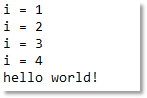7-4break测试

``````public static void main(String[] args) {
for(int i = 1; i <= 10; i++){
if(i == 5){
return;
}
System.out.println("i = " + i);
}
System.out.println("hello world!");
}``````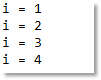7-5return测试

``````public static int method3(){
return;
}``````

``````public static void main(String[] args) {
//可以编译也可以正常运行
sumInt(10 , 20);
int retValue = sumInt(100 , 200);
System.out.println("计算结果 = " + retValue);
//编译报错，返回值类型是int，不能采用byte接收
//byte retValue2 = sumInt(1 , 2);
}
public static int sumInt(int a , int b){
return a + b;
}``````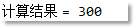7-6：测试怎么接收返回值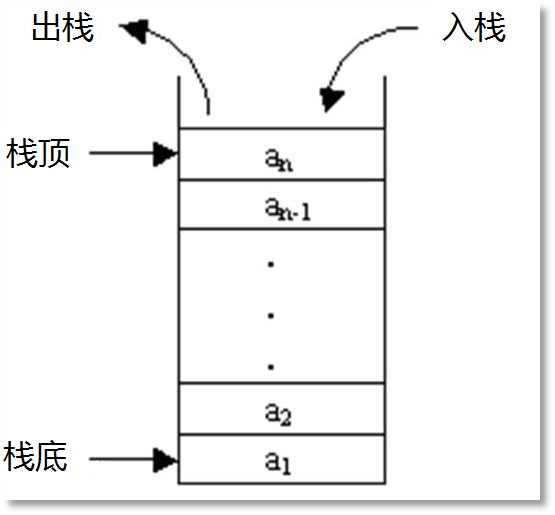7-7：栈数据结构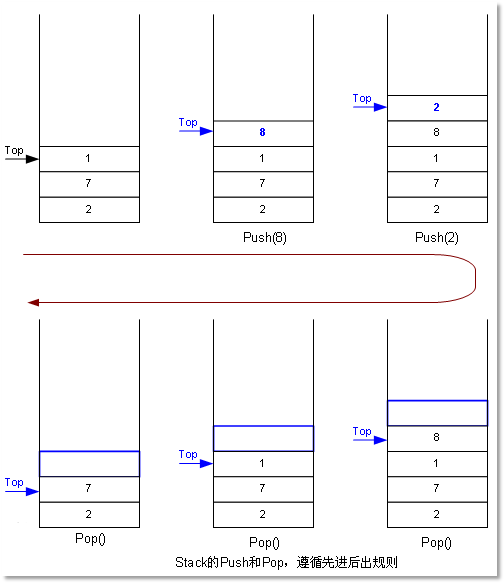7-8：栈数据结构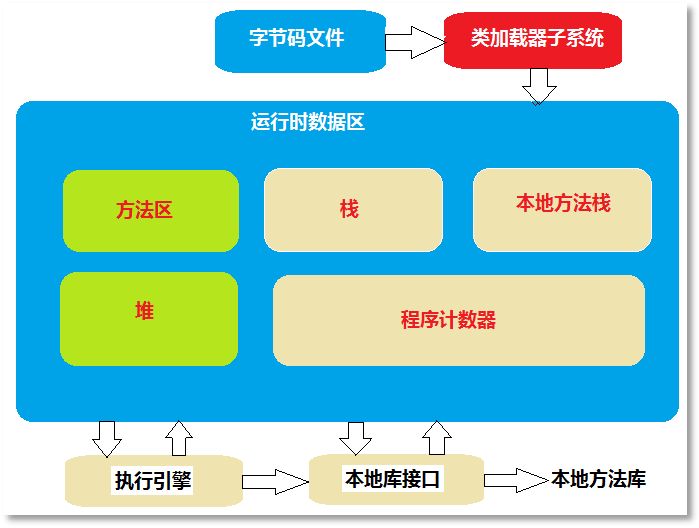7-9JVM内存结构图

``````public class MethodTest {
public static void main(String[] args) {
System.out.println("main begin");
m1();
System.out.println("main over");
}
public static void m1() {
System.out.println("m1 begin");
m2();
System.out.println("m1 over");
}
public static void m2() {
System.out.println("m2 begin");
System.out.println("m2 over");
}
}``````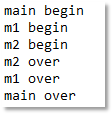7-10：方法执行过程中内存变化测试程序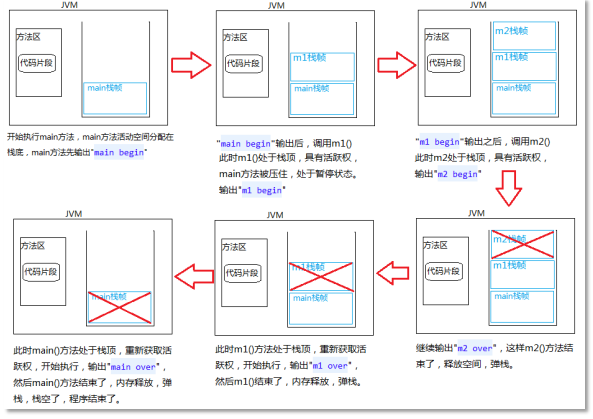7-11：方法执行过程中内存的变化

• 类加载器将class文件加载到方法区。

​​​​​​​

• 开始调用main方法，在栈内存中给main方法分配空间，开始执行main方法，输出main begin

​​​​​​​

• 调用m1()方法，在栈内存中给m1()方法分配空间，m1()方法处于栈顶，具备活跃权，输出m1 begin

​​​​​​​

• 调用m2()方法，在栈内存中给m2()方法分配空间，m2()方法处于栈顶，具备活跃权，输出m2 begin，继续输出m2 over

​​​​​​​

• m2()方法执行结束，内存释放，弹栈。

​​​​​​​

• m1()方法这时处于栈顶，具备活跃权，输出m1 over

​​​​​​​

• m1()方法执行结束，内存释放，弹栈。

​​​​​​​

• main()方法这时处于栈顶，具备活跃权，输出main over

​​​​​​​

• main()方法执行结束，内存释放，弹栈。

​​​​​​​

• 栈空了，程序结束。

​​​​​​​